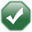# DATECALC - Date calculator (Reports & Settings Report Generator function)

## Syntax

```\$DATECALC{date, formula, N/T, CCYYMMDD}
```

## Description

Apply a formula to a date.

## Parameters

date
A numeric parameter that can be omitted.
Input date. If omitted, the current machine date is used.
formula
A text parameter that can not be omitted.
The formula to apply to the starting 'date'.

### With offset

Eg. '+1D', '1Y', '-1Y'

D
Days
M
Months
Y
Years

If you want to add a unit to the date then you should just use the number you want to add and if you want to subtract the units from the date just use a '-' before the number.

A combination of units can be used. E.g. '-1Y+1M' would subtract 1 year and then add 1 month. Of course you could just subtract 11 months ('-11M').

#### Note

If you are using the year or month units you should note if the date supplied was the last day in a month that doesn't exist in the new year calculated the last day in the new month will be used. So don't worry if you add one year to a february date that was set to the last day of that february. The 29th of february in a leap year would be converted to the 28th of february in the new calculated year, unless of course you were adding a multiple of four years to the date, in this case the 29th february would be kept.

### Without offset

Eg. '+', 'D', '='

=
User Date
.
Machine Date
-
Previous Day
+
Next Day
M
First day of the current month.
H
Last day of the current month.
Y
First day of the current year.
R
Last day of the current year.
W
First day of the current week (Mon).
K
Last day of the current week (Sun).

A combination of units can be used. E.g. '=--' would subtract 2 days from the current user date. Another way to write that would be '=-2D'.'+1D', '+' Add one day from the date supplied. '=+1D', '=+' Add one day from the user date. '=-3MH' The last day in the month three months from the user date.
N/T
A text parameter that can be omitted (it defaults to "N").
N
Numeric result. This is the number of days since the first of January 1980. This is most commonly used for sorting purposes.
T
Text result. This is the date formatted for display, e.g. '01/01/2004'. You can optionally use the next parameter to control the exact format.
CCYYMMDD
A text parameter that can be omitted (it defaults to "DD/MM/CCYY").
When the previous parameter is 'T', this parameter can be used to control the exact format. The following four values can be combined in any order, with separating characters if required.
DD
Day (01 to 31).
MM
Month (01 to 12).
CC
Century (19 or 20).
YY
Year (00 to 99).DD/MM/CCYY Same as the default. MM/DD/CCYY Output in USA format. CCYYMMDD Use for sorting into date order (would have the same effect as using the numeric result). CCYYMM Use for grouping by month.

## Returns

A text or numeric (depending on the parameters) value whose typical size is 10 characters.

Feedback
Thank you for using our Knowledge Base, we value your feedback. Did you find this article useful? 'Yes' or 'No'
Keywords AND Misspellings
date, day, month, year